# 平行光struct Light {
// vec3 position; // 使用定向光就不再需要了
vec3 direction;

vec3 ambient;
vec3 diffuse;
vec3 specular;
};
...
void main()
{
vec3 lightDir = normalize(-light.direction);
...
}


for(unsigned int i = 0; i < 10; i++)
{
glm::mat4 model;
model = glm::translate(model, cubePositions[i]);
float angle = 20.0f * i;
model = glm::rotate(model, glm::radians(angle), glm::vec3(1.0f, 0.3f, 0.5f));

glDrawArrays(GL_TRIANGLES, 0, 36);
}


lightingShader.setVec3("light.direction", -0.2f, -1.0f, -0.3f);


Important

if(lightVector.w == 0.0) // 注意浮点数据类型的误差
// 执行定向光照计算
else if(lightVector.w == 1.0)
// 根据光源的位置做光照计算（与上一节一样）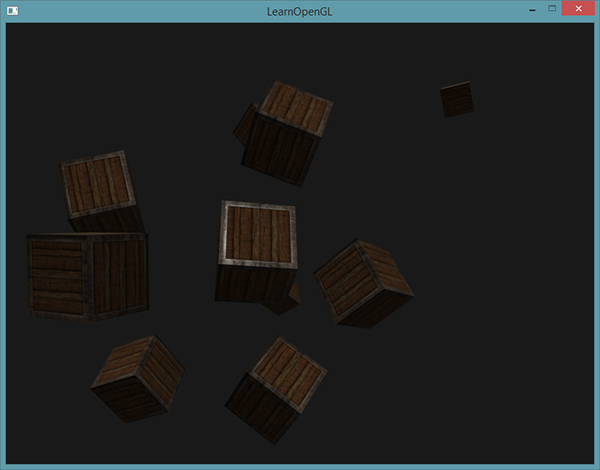# 点光源## 衰减

• 常数项通常保持为1.0，它的主要作用是保证分母永远不会比1小，否则的话在某些距离上它反而会增加强度，这肯定不是我们想要的效果。
• 一次项会与距离值相乘，以线性的方式减少强度。
• 二次项会与距离的平方相乘，让光源以二次递减的方式减少强度。二次项在距离比较小的时候影响会比一次项小很多，但当距离值比较大的时候它就会比一次项更大了。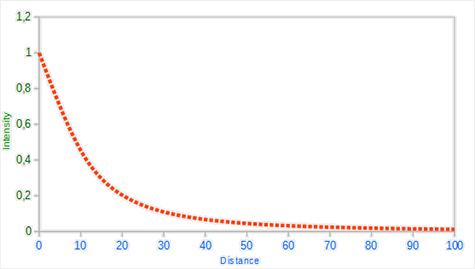### 选择正确的值

7 1.0 0.7 1.8
13 1.0 0.35 0.44
20 1.0 0.22 0.20
32 1.0 0.14 0.07
50 1.0 0.09 0.032
65 1.0 0.07 0.017
100 1.0 0.045 0.0075
160 1.0 0.027 0.0028
200 1.0 0.022 0.0019
325 1.0 0.014 0.0007
600 1.0 0.007 0.0002
3250 1.0 0.0014 0.000007

### 实现衰减

struct Light {
vec3 position;

vec3 ambient;
vec3 diffuse;
vec3 specular;

float constant;
float linear;
};


lightingShader.setFloat("light.constant",  1.0f);


float distance    = length(light.position - FragPos);
float attenuation = 1.0 / (light.constant + light.linear * distance +


Important

ambient  *= attenuation;
diffuse  *= attenuation;
specular *= attenuation;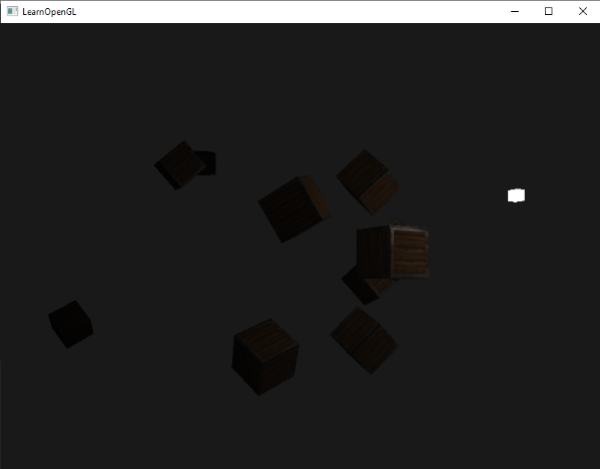# 聚光

OpenGL中聚光是用一个世界空间位置、一个方向和一个切光角(Cutoff Angle)来表示的，切光角指定了聚光的半径（译注：是圆锥的半径不是距光源距离那个半径）。对于每个片段，我们会计算片段是否位于聚光的切光方向之间（也就是在锥形内），如果是的话，我们就会相应地照亮片段。下面这张图会让你明白聚光是如何工作的：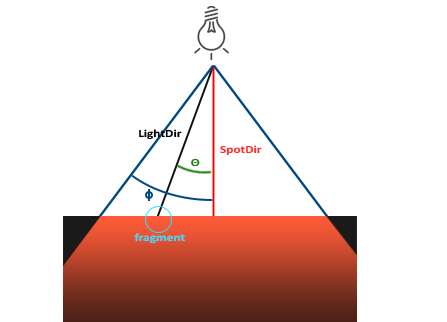• LightDir：从片段指向光源的向量。
• SpotDir：聚光所指向的方向。
• Phi$\phi$：指定了聚光半径的切光角。落在这个角度之外的物体都不会被这个聚光所照亮。
• Theta$\theta$LightDir向量和SpotDir向量之间的夹角。在聚光内部的话$\theta$值应该比$\phi$值小。

## 手电筒

struct Light {
vec3  position;
vec3  direction;
float cutOff;
...
};


lightingShader.setVec3("light.position",  camera.Position);


float theta = dot(lightDir, normalize(-light.direction));

if(theta > light.cutOff)
{
// 执行光照计算
}
else  // 否则，使用环境光，让场景在聚光之外时不至于完全黑暗
color = vec4(light.ambient * vec3(texture(material.diffuse, TexCoords)), 1.0);


Important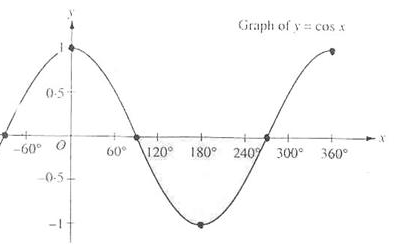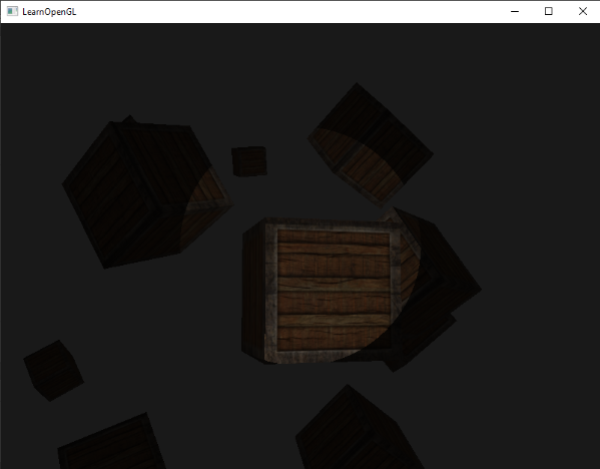## 平滑/软化边缘

$\theta$ $\theta$（角度） $\phi$（内光切） $\phi$（角度） $\gamma$（外光切） $\gamma$（角度） $\epsilon$ $I$
0.87 30 0.91 25 0.82 35 0.91 - 0.82 = 0.09 0.87 - 0.82 / 0.09 = 0.56
0.9 26 0.91 25 0.82 35 0.91 - 0.82 = 0.09 0.9 - 0.82 / 0.09 = 0.89
0.97 14 0.91 25 0.82 35 0.91 - 0.82 = 0.09 0.97 - 0.82 / 0.09 = 1.67
0.83 34 0.91 25 0.82 35 0.91 - 0.82 = 0.09 0.83 - 0.82 / 0.09 = 0.11
0.64 50 0.91 25 0.82 35 0.91 - 0.82 = 0.09 0.64 - 0.82 / 0.09 = -2.0
0.966 15 0.9978 12.5 0.953 17.5 0.9978 - 0.953 = 0.0448 0.966 - 0.953 / 0.0448 = 0.29

float theta     = dot(lightDir, normalize(-light.direction));
float epsilon   = light.cutOff - light.outerCutOff;
float intensity = clamp((theta - light.outerCutOff) / epsilon, 0.0, 1.0);
...
// 将不对环境光做出影响，让它总是能有一点光
diffuse  *= intensity;
specular *= intensity;
...## 练习

• 尝试实验一下上面的所有光照类型和它们的片段着色器。试着对一些向量进行取反，并使用 < 来代替 >。试着解释不同视觉效果产生的原因。